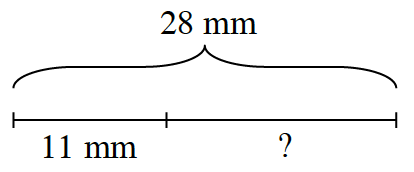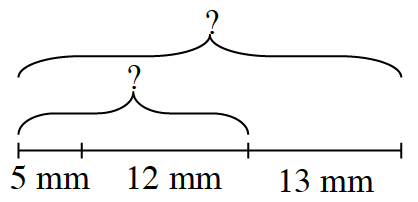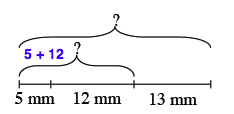### Home > CC2 > Chapter Ch1 > Lesson 1.1.1 > Problem1-5

1-5.

According to the diagrams below, how long is each unknown piece?

1.How long is the entire length?
Which part is $11$ mm?
Try to answer the question with these things in mind.

$11+?=28$

How long is the unknown length?

$17$ mm, because $11 + 17 = 28$.

1.How long is the shorter unknown length?The shorter length is $17$ mm. Explain why on your paper.

Now use the same method to find the entire length.